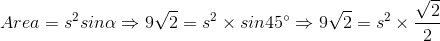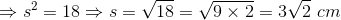# ISEE Upper Level Math : How to find the length of the side of a rhombus

## Example Questions

### Example Question #4 : Rhombuses

A rhombus has a perimeter of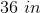. Give the side length of the rhombus.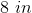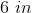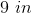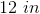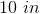Explanation:

Like any polygon, the perimeter of the rhombus is the total distance around the outside, which can be found by adding together the length of each side. In the case of a rhombus, all four sides have the same length, i.e.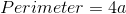,

whereis the length of each side.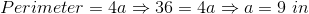### Example Question #5 : Rhombuses

A rhombus has the area of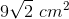. One of the interior angles is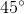. Give the length of each side of the rhombus.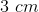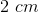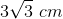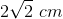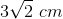Explanation:

The area of a rhombus can be determined by the following formula: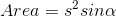,

whereis the length of any side and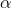is any interior angle.